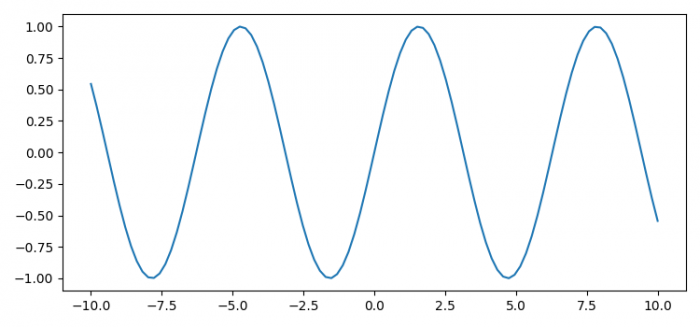# How to show a figure that has been closed in Matplotlib?

To show a figure that has been closed in Matplotlib, we can create a new Canvas Manager and store the previous figure into a new Canvas figure.

## Steps

• Set the figure size and adjust the padding between and around the subplots.
• Create a new figure or activate an existing figure.
• Create x and y data points using numpy.
• Plot x and y data points using plot() method.
• Close the current figure where the plot has been plotted.
• Now, store the previous figure in a new Canvas figure.
• Set the Canvas that contains the figure.
• To display the figure, use show() method.

## Example

import numpy as np
from matplotlib import pyplot as plt

plt.rcParams["figure.figsize"] = [7.50, 3.50]
plt.rcParams["figure.autolayout"] = True

fig = plt.figure()
x = np.linspace(-10, 10, 100)
y = np.sin(x)

plt.plot(x, y)
plt.close(fig)

new_fig = plt.figure()
new_manager = new_fig.canvas.manager
new_manager.canvas.figure = fig
fig.set_canvas(new_manager.canvas)

plt.show()

## Output